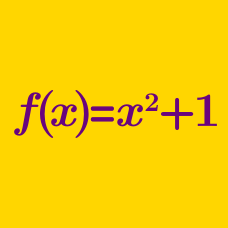Algebra

# Composition of Functions

Given two functions:

$$f(x)=x+3$$

$$g(x)=\dfrac{x}{2}$$

Find the value of $$g(f(5))$$.

For the two functions $f(x)=x^2+5x, \, g(x)=x+22,$ what is the value of $$(g \circ f)(9)?$$

Details and assumptions

$$(g \circ f)(x)$$ is the composition of $$g$$ and $$f$$, defined by $$(g \circ f)(x)= g(f(x))$$.

Let $$f$$ be a function such that $$(f \circ f)(x) = x^2$$

What is the value of $$f(f(f(f(f(f(2))))))?$$

Consider the two functions $f(x)=6x-5, g(x)=\begin{cases} -x+4 & \text{ if } x \geq 1, \\ 17 & \text{ if } x<1. \end{cases}$ What is the value of $$(f \circ g)(2)+(g \circ f)(0)$$?

Details and assumptions

$$(g \circ f)(x)$$ is the composition of $$g$$ and $$f$$, defined by $$(g \circ f)(x)= g(f(x))$$.

$$f: \mathbb{R} \rightarrow \mathbb{R}$$ is a function satisfying $$f( 2x+1) = 4x^2 + 4x$$. What is the value of $$f(16)$$?

×